• 问答
• 技术
• 实践
• 资源

## 本文目录

19 LeViT: 用于快速推理的视觉Transformer
(来自 Facebook，DeiT 一作 Hugo Touvron 挂名)
19.1 LeViT原理分析

20 ViT-Lite: 紧凑型视觉Transformer，更小更简单
(来自 俄勒冈大学，UIUC，PAIR)
20.1 ViT-Lite原理分析

Transformer 是 Google 的团队在 2017 年提出的一种 NLP 经典模型，现在比较火热的 Bert 也是基于 Transformer。Transformer 模型使用了 Self-Attention 机制，不采用 RNN 的顺序结构，使得模型可以并行化训练，而且能够拥有全局信息。

## 19 LeViT：用于快速推理的视觉Transformer

https://arxiv.org/pdf/2104.01136.pdf​arxiv.org

• 19.1 LeViT原理分析：

Training data-efficient image transformers \& distillation through attention​arxiv.org

### DeiT

DeiT可以通过一组很优秀的超参数使得在不改变任何架构的前提下实现了ViT的涨点。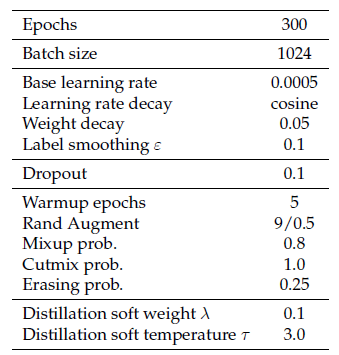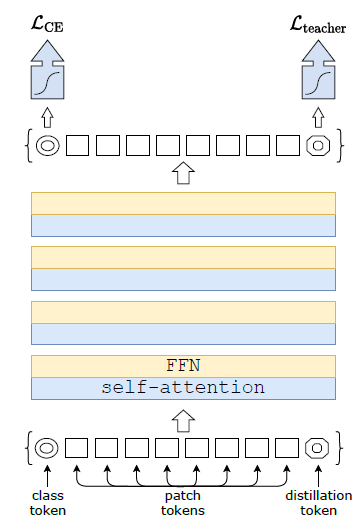ViT的输出是一个softmax，它代表着预测结果属于各个类别的概率的分布。ViT的做法是直接将这个softmax与GT label取 $\text{CE Loss}$ 。

$$\begin{equation} \mathcal{L}\mathrm{global} = (1-\lambda) \mathcal{L}\mathrm{CE}(\psi(Z\mathrm{s}),y) + \lambda \tau^2 \mathrm{KL}(\psi(Z\mathrm{s}/\tau),\psi(Z_\mathrm{t}/\tau)) \end{equation} \tag{19.1}$$

$$\begin{equation} \mathcal{L}\mathrm{global}^\mathrm{hard Distill} = \frac{1}{2}\mathcal{L}\mathrm{CE}(\psi(Zs),y) + \frac{1}{2}\mathcal{L}\mathrm{CE}(\psi(Zs),y\mathrm{t}) \end{equation}\ y_\mathrm{t}=\mathrm{argmax}c Z\mathrm{t}(c) \tag{19.2}$$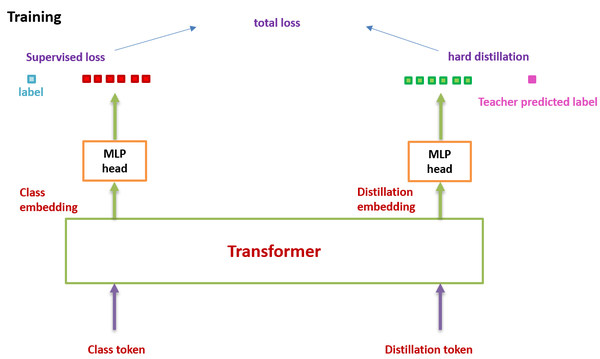### LeViT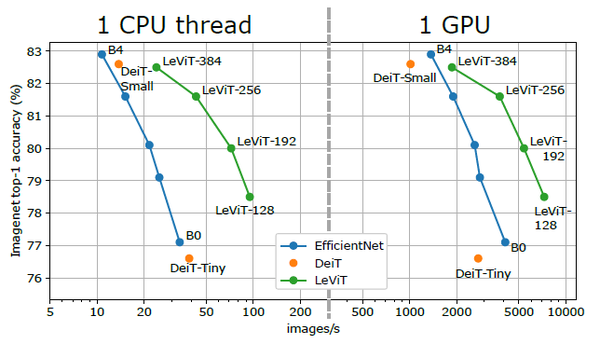LeViT 的实现方案又重新引入了卷积操作，做成了一个类似于CNN中的LeNet的架构，因此称之为LeViT，LeViT的技术可以使得ViT模型在width和spatial resolution上面更小。那么LeViT为甚么要引入卷积操作呢？这来自于作者一开始做的一个把CNN和Transformer结构嫁接的实验：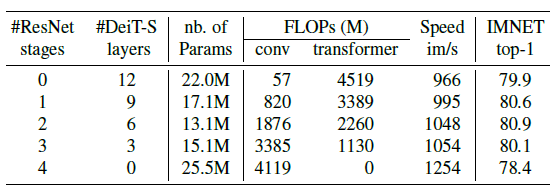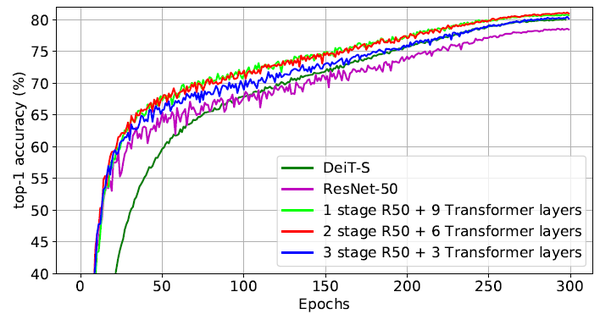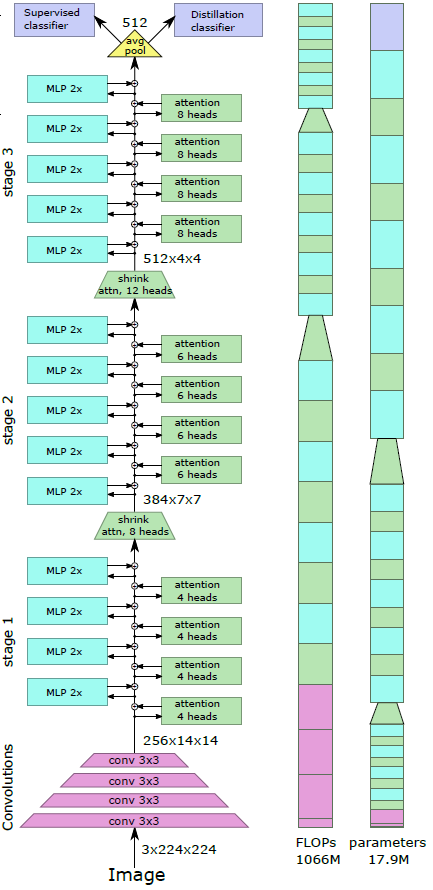1 借助卷积提特征

2 不使用class token

3 归一化层和激活函数

LeViT中每个卷积层之后紧跟一个Batch Normalization层，所有的激活函数都使用了GELU函数。

4 LeViT attention blocks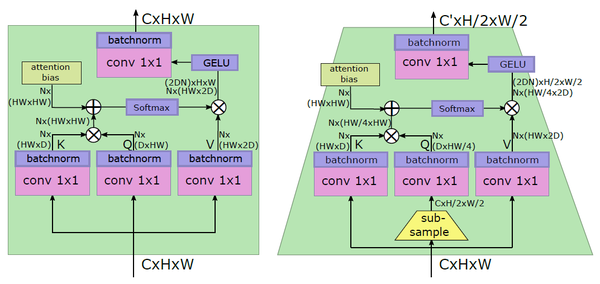5 用Attention bias取代位置编码

LeViT去掉了ViT/DeiT中的传统的位置编码方式，作者认为不仅是输入阶段的位置编码，中间层保持位置信息也同样重要。LeViT实现位置编码的方法是在attention map中引入了bias，就是下式的第2项的 $B$ 这个张量。它的每个head有 $H\times W$ 个值，对应于不同的偏置距离，绝对值使得这种位置编码方式编码的是一种相对距离，例如 $Q{(1,3)}$ 和 $K{(2,5)}$ 对应 $B^H{1,2}$ ，而 $Q{(3,7)}$ 和 $K{(2,5)}$ 也对应 $B^H{1,2}$。

$$\begin{equation} A^h{(x, y), (x', y')} = Q{(x,y),:}\bullet K{(x',y'),:} + B^h{|x-x'|,|y-y'|}. \end{equation} \tag{19.3}$$

6 LeViT中的MLP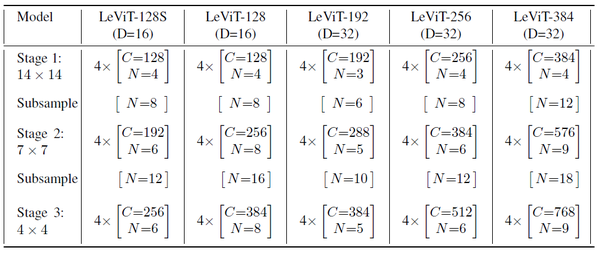### Experiments：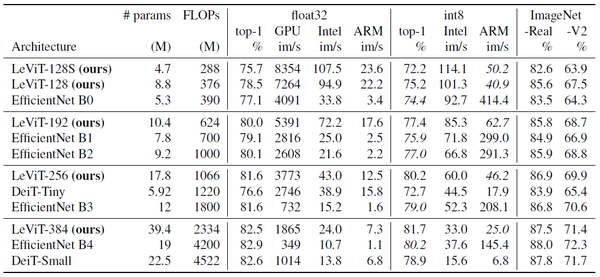LeViT-384的准确率与DeiT-Small很接近，但是计算量仅为后者的一半。

LeViT-128的准确率比DeiT-Tiny更高，但是计算量仅为后者三分之一。

LeViT-128，LeViT-256的精度与EfficientNet B2，B3很接近，但是在CPU上的速度却分别快了2.8倍和3.3倍。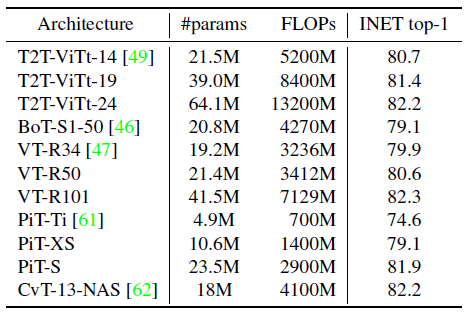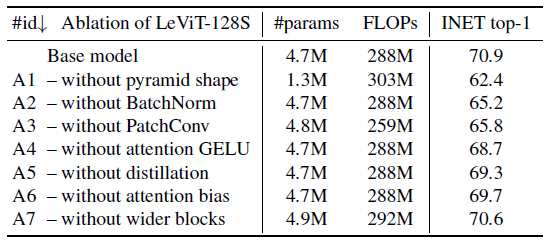A1： 抛弃金字塔结构，即：随着层数的加深，空间分辨率递减，dimension递增的操作。为了使得计算量和baseline尽量接近，就减少width。

A2： 把BatchNorm换成LayerNorm，性能稍有掉点。

A3： 去掉一开始的4层卷积，对性能影响很大。因为对于Low-level的信息，卷积操作还是最好的手段。

A4： 去掉GELU损失函数，即减少模型的non-linearity，出现了掉点，证明非线性也有一定的作用。

A5： 不使用蒸馏损失，也会掉点。但是由于卷积的补偿作用，掉点比DeiT少很多。

A6： 把attention bias 换成传统的不可学习位置编码：attention bias在LeViT中充当了位置编码的作用，换成另一种位置编码影响不大。

## 小结：

LeViT可以看做是另一个对DeiT的改进，主要考虑提升模型的前向推理速度。它的主要手段是：

• 融合卷积，提取底层特征。
• 把Linear Transformation的操作全部都用Conv 1×1 + BatchNorm实现。
• 使用金字塔结构。
• 去掉class token。
• 使用attention bias取代传统的不可学习位置编码。

## 20 ViT-Lite: 紧凑型视觉Transformer，更小更简单

Escaping the Big Data Paradigm with Compact Transformers​arxiv.org

• 20.1 ViT-Lite原理分析：

Transformers lack some of the inductive biases inherent to CNNs, such as translation equivariance and locality, and therefore do not generalize well when trained on insufficient amounts of data.

### CvT：

CvT首先embedding的方式变成了卷积操作，在每个Multi-head self-attention之前都进行Convolutional Token Embedding。其次在 Self-attention的Projection操作不再使用传统的Linear Projection，而是使用Convolutional Projection。

### TNT：

TNT建模每个patch的内部信息，通过将Patch内部信息与Patch之间的信息融合，提升了DeiT/ViT的性能。

### ConViT：

https://arxiv.org/pdf/2103.10697.pdf​arxiv.org

ViT-Lite几乎和ViT一模一样，只是对于小数据集来说使用了更小的patch，作者认为针对不同量级的数据集，最适合的patch大小是不同的，这点很重要。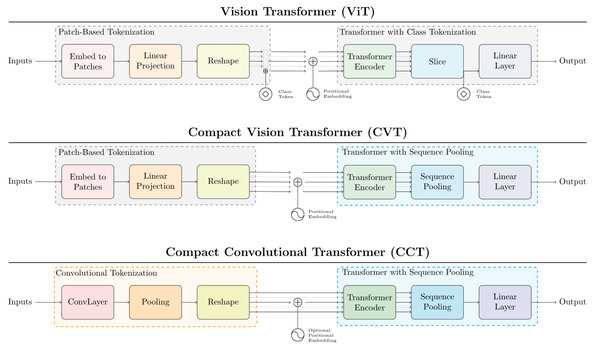### 从ViT变成CVT

CVT在ViT的基础上引入了一个称为SeqPool的池化方法，该方法将Transformer Encoder产生的基于顺序的信息池化。

SeqPool的池化方法将整个序列的数据集中起来， 我们可以把这个操作看成是映射变换 $T: \mathbb{R}^{b \times n \times d} \mapsto \mathbb{R}^{b \times d}$ ：

$$\begin{equation} {x}_L = f({x}_0) \in \mathbb{R}^{b \times n \times d} \end{equation} \tag{20.1}$$

$$\begin{equation} {x}_L^{\prime} = {softmax}\left({g}({x}_L)^{T}\right) \in{R}^{b \times 1 \times n} \end{equation} \tag{20.2}$$

$$\begin{equation} {z} = {x}_L^{\prime} {x}_L = {softmax}\left({g}({x}_L)^{T}\right) \times {x}_L \in {R}^{b \times 1 \times d} \end{equation} \tag{20.3}$$

SeqPool这个操作相当于是允许我们对Encoder输出的 $n$ 个维度进行加权平均，对于序列数据进行加权得到最终的结果。

### 从CVT变成CCT

CCT为了给模型引入inductive bias，在给图片分patch的环节使用了卷积层。比如输入图片 $x\in R^{H\times W\times C}$ ：

$$x_0=\text{MaxPool}(ReLU(Conv2d(x))) \tag{20.4}$$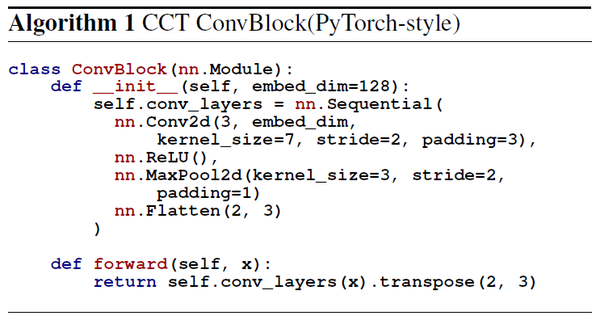CCT和CVT的区别如下图15所示：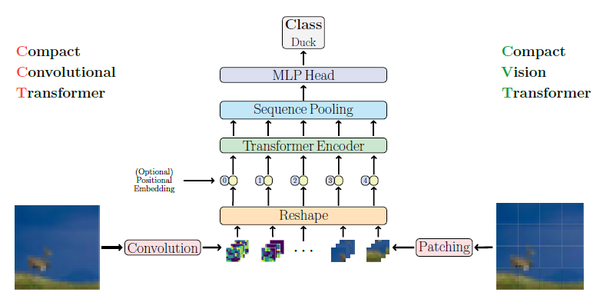### Experiments：

Epochs 200
batch size 128
Learning rate schedule cosine annealing
warm up epochs 5 or 10

### Performance Comparison

ViT12/8代表12层的ViT模型，patch大小为8。

CCT 6/3代表图片分patch的环节使用6个卷积堆叠，卷积核大小是3×3的。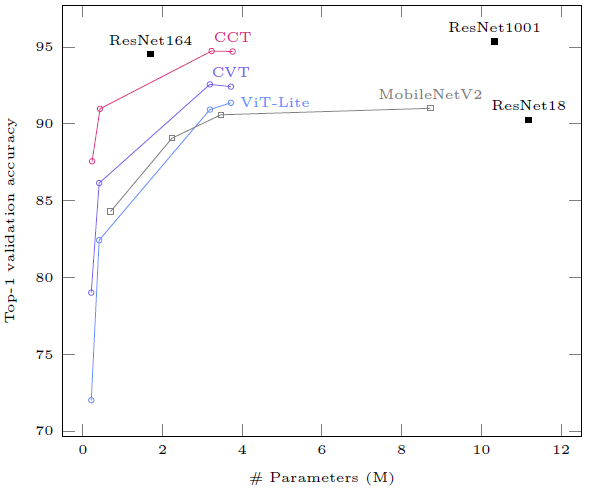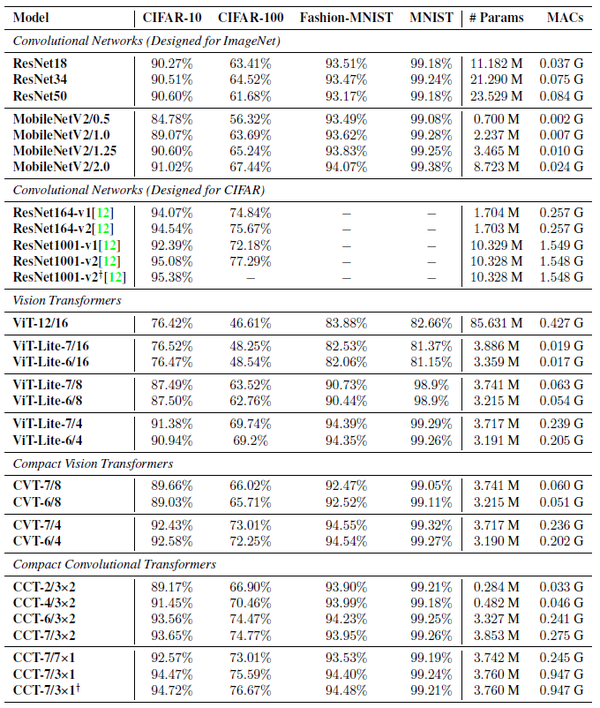Pool这一列代表使用的池化方法：CT代表不使用池化，而继续使用class token；SP代表Sequence Pooling。

Conv代表是否在图片分patch的环节使用卷积。

Tuning代表对于dropout rate等超参数做微小的改变，不使用MLP dropout，只使用attention dropout。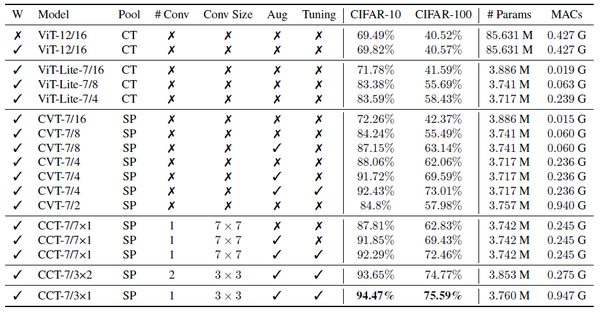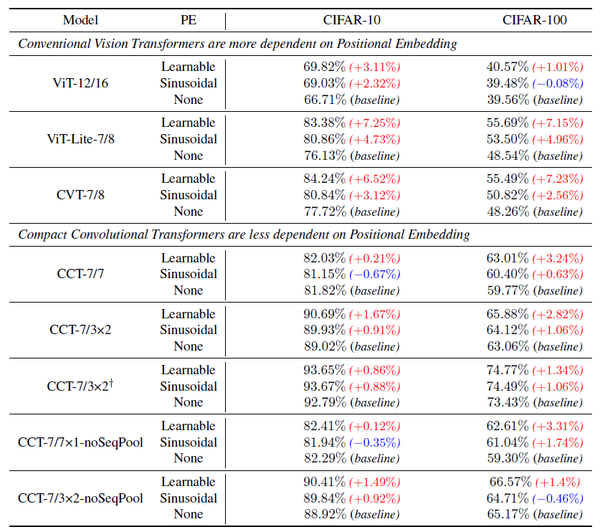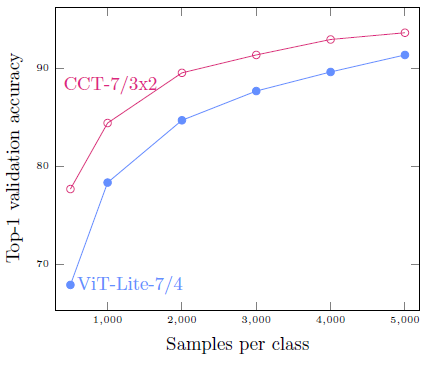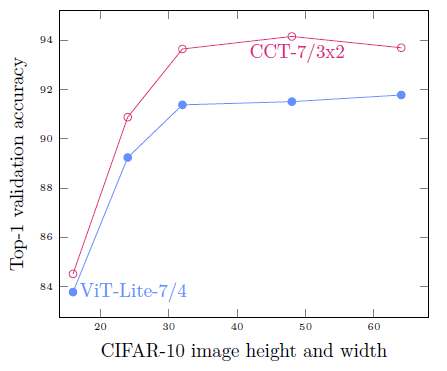## 总结：

•0
•0
•96收藏

• 13,409

关注
• 283

获赞
• 50

精选文章

• 从事AI视觉算法开发多年，主攻目标检测、图像分割方向

• 优质论文推荐

• 46
• 148
• 132
• 65
• 96